NCERT Solutions for Class 8 Maths Chapter 11 Mensuration Ex 11.4 are part of NCERT Solutions for Class 8 Maths. Here we have given NCERT Solutions for Class 8 Maths Chapter 11 Mensuration Ex 11.4.

 Board CBSE Textbook NCERT Class Class 8 Subject Maths Chapter Chapter 11 Chapter Name Mensuration Exercise Ex 11.4 Number of Questions Solved 8 Category NCERT Solutions

## NCERT Solutions for Class 8 Maths Chapter 11 Mensuration Ex 11.4

Question 1.
Given a cylindrical tank, in which situation will you find the surface area and in which situation volume?
(a) To find how much it can hold.
(b) Number of cement bags required to plaster it.
(c) To find the number of smaller tanks that can be filled with water from it.
Solution.
(a) Volume
(b) Surface area
(c) Volume.

Question 2.
The diameter of cylinder A is 7 cm, and the height is 14 cm. The diameter of cylinder B is 14 cm and height is 7 cm. Without doing any calculations can you suggest whose volume is greater? Verify it by finding the volume of both the cylinders. Check whether the cylinder with greater volume also has a greater surface area?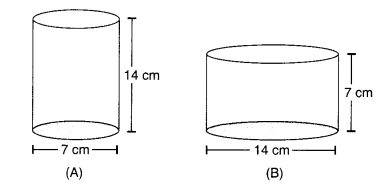Solution.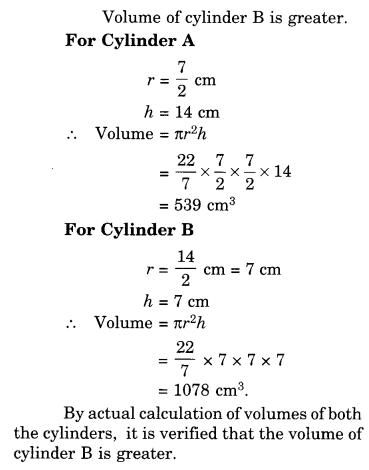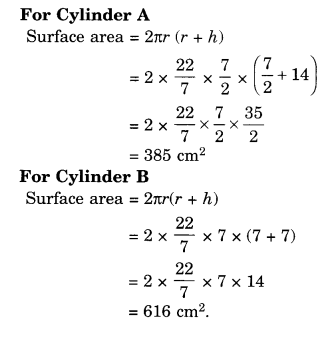Question 3.
Find the height of a cuboid whose base area is 180 $${ cm }^{ 2 }$$ and volume is 900 $${ cm }^{ 3 }$$.
Solution.
Height of the cuboid
= $$\frac { Volume\quad of\quad the\quad cuboid }{ Base\quad area\quad of\quad the\quad cuboid }$$
= $$\frac { 900 }{ 180 }$$
= 5 cm

Question 4.
A cuboid is of dimensions 60 cm x 54 cm x 30 cm. How many small cubes with side 6 cm can be placed in the given cuboid?
Solution.
A volume of the cuboid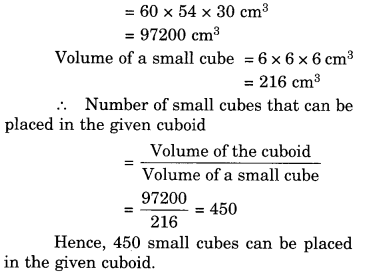Question 5.
Find the height of the cylinder whose volume is 1.54 $${ m }^{ 3 }$$ and diameter of the base is 140 cm.
Solution.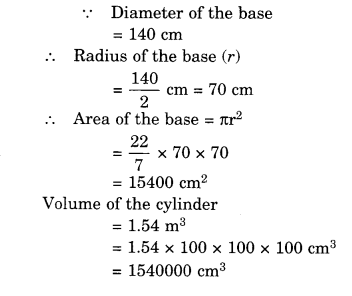Question 6.
A milk tank is in the form of a cylinder whose radius is 1.5 m and length is 7 m. Find the quantity of milk in liters that can be stored in the tank.
Solution.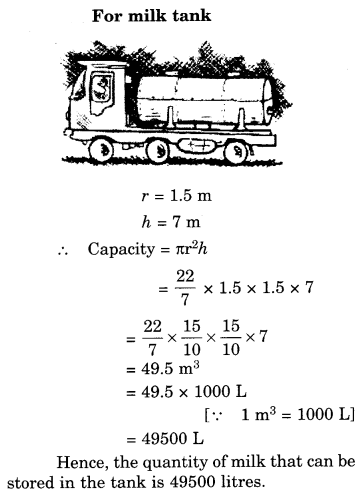Question 7.
If each edge of a cube is doubled,
(i) how many times will its surface area increase?
(ii) how many times will its volume increase?
Solution.
Let the original edge of the cube be a cm.
Then, its new edge = 2a cm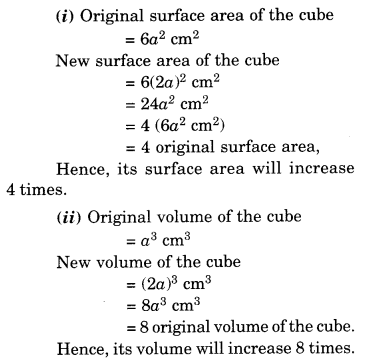Question 8.
Water is pouring into a cuboidal reservoir at the rate of 60 liters per minute. If the volume of the reservoir is 108 m3, find the number of hours it will take to fill the reservoir.
Solution.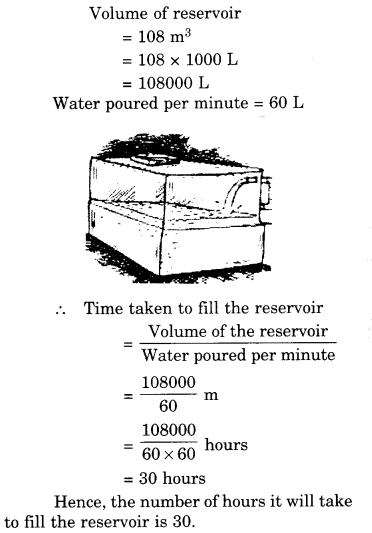We hope the NCERT Solutions for Class 8 Maths Chapter 11 Mensuration Ex 11.4 help you. If you have any query regarding NCERT Solutions for Class 8 Maths Chapter 11 Mensuration Ex 11.4, drop a comment below and we will get back to you at the earliest.